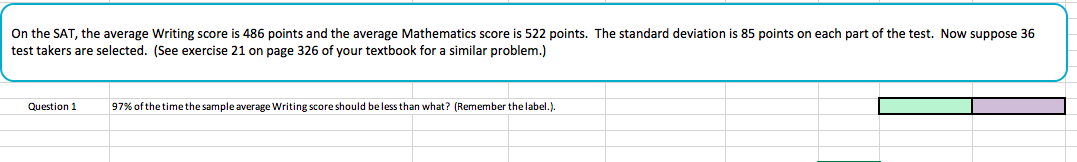# On the SAT, the average Writing score is 486 points and the average Mathematics score is 522 points. The standard deviation is 85 points on each part of the test. Now suppose 36 test takers are selected. (See exercise 21 on page 326 of your textbook for a similar problem.) Question 97% of the time the sample average writing score should be less than what? (Remember the label.),

Questionhelp_outlineImage TranscriptioncloseOn the SAT, the average Writing score is 486 points and the average Mathematics score is 522 points. The standard deviation is 85 points on each part of the test. Now suppose 36 test takers are selected. (See exercise 21 on page 326 of your textbook for a similar problem.) Question 97% of the time the sample average writing score should be less than what? (Remember the label.), fullscreen

### Want to see this answer and more?

Experts are waiting 24/7 to provide step-by-step solutions in as fast as 30 minutes!*